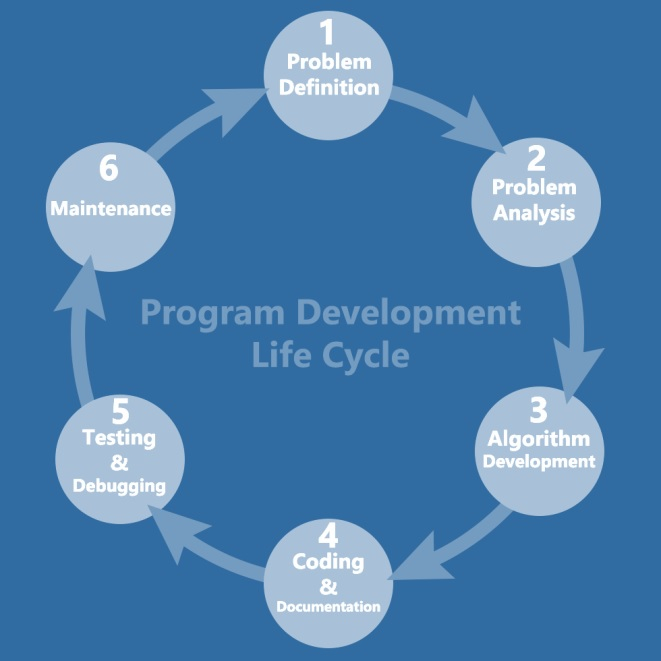# What is program development cycle in C language?

When we want to develop a program by using any programming language, we have to follow a sequence of steps. These steps are called phases in program development.

The program development life cycle is a set of steps or phases which are used to develop a program in any programming language.

## Phases of program development

Program development life cycle contains 6 phases, which are as follows −

• Problem Definition.
• Problem Analysis.
• Algorithm Development.
• Coding & Documentation.
• Testing & Debugging.
• Maintenance.

These six phases are depicted in the diagram given below −## Problem Definition

Here, we define the problem statement and decide the boundaries of the problem.

In this phase, we need to understand what is the problem statement, what is our requirement and what is the output of the problem solution. All these are included in the first phase of program development life cycle.

## Problem Analysis

Here, we determine the requirements like variables, functions, etc. to solve the problem. It means that we gather the required resources to solve the problem, which are defined in the problem definition phase. Here, we also determine the bounds of the solution.

## Algorithm Development

Here, we develop a step-by-step procedure that is used to solve the problem by using the specification given in the previous phase. It is very important phase for the program development. We write the solution in step-by-step statements.

## Coding & Documentation

Here, we use a programming language to write or implement the actual programming instructions for the steps defined in the previous phase. We construct the actual program in this phase. We write the program to solve the given problem by using the programming languages like C, C++, Java, etc.

## Testing & Debugging

In this phase, we check whether the written code in the previous step is solving the specified problem or not. This means, we try to test the program whether it is solving the problem for various input data values or not. We also test if it is providing the desired output or not.

## Maintenance

In this phase, we make the enhancements. Therefore, the solution is used by the end-user. If the user gets any problem or wants any enhancement, then we need to repeat all these phases from the starting, so that the encountered problem is solved or enhancement is added.